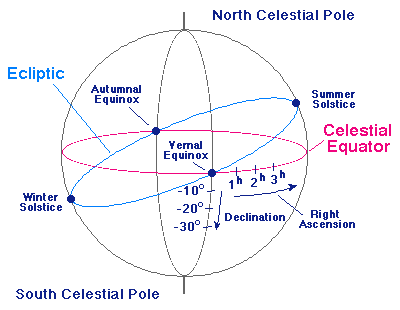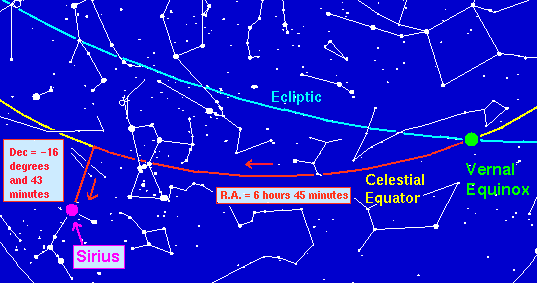Celestial Coordinate
System

It is useful to impose on the celestial sphere a coordinate system that is analogous to the latitude-longitude system employed for the surface of the Earth.

## Right Ascension and Declination

This coordinate system is illustrated in the following figure (for which you should imagine the earth to be a point at the center of the sphere).The celestial coordinate system

In the celestial coordinate system the North and South Celestial Poles are determined by projecting the rotation axis of the Earth to intersect the celestial sphere, which in turn defines a Celestial Equator. The celestial equivalent of latitude is called declination and is measured in degrees North (positive numbers) or South (negative numbers) of the Celestial Equator. The celestial equivalent of longitude is called right ascension. Right ascension can be measured in degrees, but for historical reasons it is more common to measure it in time (hours, minutes, seconds): the sky turns 360 degrees in 24 hours and therefore it must turn 15 degrees every hour; thus, 1 hour of right ascension is equivalent to 15 degrees of (apparent) sky rotation.

## Equinoxes and Solstices

The zero point for celestial longitude (that is, for right ascension) is the Vernal Equinox, which is that intersection of the ecliptic and the celestial equator near where the Sun is located in the Northern Hemisphere Spring. The other intersection of the Celestial Equator and the Ecliptic is termed the Autumnal Equinox. When the Sun is at one of the equinoxes the lengths of day and night are equivalent (equinox derives from a root meaning "equal night"). The time of the Vernal Equinox is typically about March 21 and of the Autumnal Equinox about September 22.

The point on the ecliptic where the Sun is most north of the celestial equator is termed the Summer Solstice and the point where it is most south of the celestial equator is termed the Winter Solstice. In the Northern Hemisphere the hours of daylight are longest when the Sun is near the Summer Solstice (around June 22) and shortest when the Sun is near the Winter Solstice (around December 22). The opposite is true in the Southern Hemisphere. The term solstice derives from a root that means to "stand still"; at the solstices the Sun reaches its most northern or most southern position in the sky and begins to move back toward the celestial equator. Thus, it "stands still" with respect to its apparent North-South drift on the celestial sphere at that time.

Traditionally, Northern Hemisphere Spring and Fall begin at the times of the corresponding equinoxes, while Northern Hemisphere Winter and Summer begin at the corresponding solstices. In the Southern Hemisphere, the seasons are reversed (e.g., Southern Hemisphere Spring begins at the time of the Autumnal Equinox).

## Coordinates on the Celestial Sphere

The right ascension (R.A.) and declination (dec) of an object on the celestial sphere specify its position uniquely, just as the latitude and longitude of an object on the Earth's surface define a unique location. Thus, for example, the star Sirius has celestial coordinates 6 hr 45 min R.A. and -16 degrees 43 minutes declination, as illustrated in the following figure.Right Ascension and Declination for Sirius

This tells us that when the vernal equinox is on our celestial meridian, it will be 6 hours and 45 minutes before Sirius crosses our celestial meridian, and also that Sirius is a little more than 16 degrees South of the Celestial Equator.

## Keeping your Perspective

Do not become confused because the perspectives in the celestial sphere diagram and the sky segment diagram containing Sirius are different. In the celestial sphere diagram one is imagining an outside view of the celestial sphere (from a vantage point beyond the most distant stars that we see with the naked eye). In the diagram showing the position of Sirius in the sky the view is instead the actual sky as viewed from the Earth (that is, from the center of the sphere in the first diagram).

Thus, the directions get reversed: moving to the right from the vernal equinox in the first diagram will look like moving to the left as viewed from its center, which is the perspective of the second diagram (that is, the actual view of the sky from Earth). That direction, by convention, is chosen to be the positive direction for right ascension.